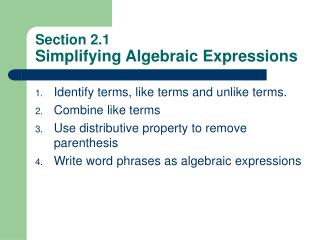DownloadDownload PresentationSection 2.1 Simplifying Algebraic Expressions

# Section 2.1 Simplifying Algebraic Expressions

Download Presentation## Section 2.1 Simplifying Algebraic Expressions

- - - - - - - - - - - - - - - - - - - - - - - - - - - E N D - - - - - - - - - - - - - - - - - - - - - - - - - - -
##### Presentation Transcript

1. Section 2.1Simplifying Algebraic Expressions • Identify terms, like terms and unlike terms. • Combine like terms • Use distributive property to remove parenthesis • Write word phrases as algebraic expressions

2. TERMS: a number or product of numbers and variables. COEFFICIENT: the remaining factors of the term NUMERICAL COEFFICIENT: the number part of the term Definitions

3. 1,2 Using Algebra Tiles • Using algebra tiles is one way to add terms Try

4. 3. Distributive property • a(b+c)=ab+ac • Simplify 2(3x-5)+1 Try • 8-(7x+2)+3x • -2(4x-3)+2(x-5)

5. 4. Translations Try • Twice a number added to 6 • The sum of twice a number, three times the number and five times the number • The difference of seven and a number, divided by 3

6. Section 2.2The Addition Property of Equations • Define linear equation in one variable and equivalent equations. • Use the addition property to solve linear equations. • Write word phrases as algebraic expressions

7. 1. Linear Equations • Linear comes form the word line, which we will see when we get to graphing. • In a linear equation, there is one variable with no exponents. Mult, add, sub OK but no divide • Linear eq. • Not linear eq.

8. 1. Linear Equations (cont.) • EQUIVALENT EQUATIONS: are equations that that are derived by appropriate methods that have the same solution. • It will turn out that the sequence of equations that we will get when solving an equations are all equivalent.

9. 2. Addition Property • If two things are equal and I add the same thing to both, I still have two things that are equal. • It also works for subtraction. • We use the addition property to solve equations.

10. 2. Addition Property (cont.) • Example • Try

11. 3. Translations • Try The sum of two numbers is 5. • If one number is 3 find the other number • If one number is 1 find the other number • If one number is x find the other number

12. 2.3The Multiplication Property of Equality • Use the multiplication property to solve linear equations. • Use both addition and multiplication properties to solve linear equations • Write word phrases as algebraic expressions

13. 1. Multiplication Property • You can multiply (or divide) each side by the same thing and still have • Examples

14. 2. Combine Mult and Add Props • Example • Try

15. 3. Translations • If x is the first of two consecutive integers, express the sum of the two integers in terms of x • Try: if x is the sum of three consecutive odd integers, express the sum of three integers in terms of x.

16. 2.4 Solving Linear Equations • Apply the general strategy for solving linear equations. • Solve equations with fractions. • Solve equations with decimals. • Recognize identities and equations with no solutions. • Write sentences as equations and solve.

17. Solving Linear Equations in One Variable • Multiply on both sides by the LCD to clear the equation of fractions if they occur. • Use the distributive property to remove parenthesis if they occur. • Simplify each side of the equation by combining like terms.

18. Solving Linear Equations in One Variable (Cont.) • Get all variable terms on one side and all numbers on the other side by using the addition property of equality. • Get the variable alone by using the multiplication property of equality. • Check the solution by substituting it into the original equation.

19. Examples Try

20. 4. Identities • Identities are equations for which each real number is a solution • Some equations have no solutions, there are no numbers that make them true

21. 5. Translations Try • Twice a number added to seven is the same as three subtracted from the number. What is the number?

22. 2.5 An Introduction to Problem Solving • Apply steps for problem solving

23. Steps for Problem Solving • UNDERSTAND the problem. During this step become comfortable with the problem. Some ways to do this are. • Read and reread the problem. • Choose a variable to represent the unknown. • Construct a drawing. • TRANSLATE the problem into an equation. • SOLVE the equation. • INTERPRET the results. Check the proposed solution in the stated problem and state and state your conclusion.

24. Examples • The sum of four times a number and 2/3 is equal to the difference of five times the the number and 5/6. Find the number. • A 21-foot beam is to be divided so that the longer piece is 1 foot more than 3 times the shorter piece. If x represents the length of the shorter piece, find the length of both pieces • To make an international telephone call, you need the code for the country you are calling. The codes for Mali republic, Cote d’Ivoire and Niger are three consecutive odd integers whose sum is 675. Find the code for each country.